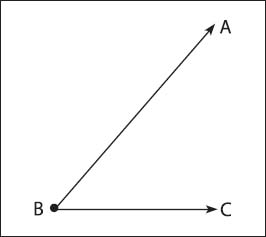NextPrevious

# What is an angle?

An angle is a major concept in both geometry and trigonometry. An angle is formed by two rays that begin at the same point; a straight angle is one in which the two rays lie on the same line. It can also be described as two planes coming from a common line.

Angles are named in several ways: a capital letter at its vertex (the common points for both rays, see illustration, ∠B), a small letter within the angle, a number within the angle, or by three capital letters (ABC or CBA, with the middle letter representing the vertex and the other two points the rays). The two rays that form the angle are called the sides of the angle, such as side BA and side BC.Angle ABC is formed by rays BA and BC linked at point B.

Close

This is a web preview of the "The Handy Math Answer Book" app. Many features only work on your mobile device. If you like what you see, we hope you will consider buying. Get the App